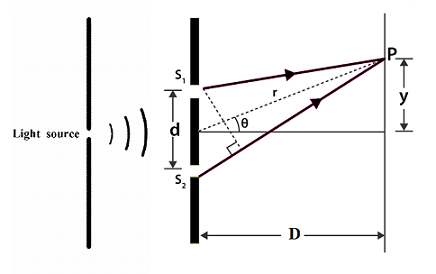# A coherent light of wavelength \lambda is incident on a double slit apparatus. Suppose that thee...

## Question:

A coherent light of wavelength {eq}\lambda{/eq} is incident on a double-slit apparatus. Suppose that the light intensity passing through one slit is dimmer by a factor of two compared to the other slit(due to a filter or attenuator). Determine the intensity {eq}\displaystyle {I_{total}}{/eq} as a function of angular position {eq}\theta{/eq} on the screen for coherent light.

## Young's Double-Slit Experiment With Asymmetric Slits:

There are two fundamental ways in which the intensity of light adds up. One is called coherent addition and the other is called incoherent addition. In the former, the waves add with a fixed phase difference. This is what is done in Young's double-slit experiment. In the latter, random phases wash out interference effects. This type of light addition is what we encounter in everyday life.

Coherent addition leads to characteristic interference patterns with regions of alternate brightness and darkness. By studying this pattern, it is possible to determine the wavelength of the incident radiation.

Let:

• the slit separation be d
• the separation between the slit plate and the screen be D
• the position of the field point on the screen be y (see figure below)It is given that the intensities are in the ratio 2:1. Let us choose them to be 2I and I. The intensity is proportional to the square of the amplitude.The amplitudes are therefore in the ratio {eq}\displaystyle {\sqrt2}{/eq}:1.

The total amplitude at a point on the screen will be the phasor sum of the two electric field phasors incident from the slits. Phasor addition is done just like vector addition but with the angle replaced by the phase difference. So we can use the parallelogram law of addition:

{eq}\displaystyle {a_{total}=\sqrt{a_{1}^{2}+a_{2}^{2}+2a_{1}a_{2}\cos\phi }}{/eq}, where:

• {eq}\displaystyle {\phi}{/eq} is the phase difference.

Squaring the above relation, we get the corresponding relation between the intensities:

The phase difference between the two electromagnetic waves will be a function of the path difference. Now the path difference is given by:

{eq}\displaystyle {S_{2}P-S_{1}P=\frac{yd}{D}}{/eq}, where:

• P is the point of interest located on the screen.

A path difference of {eq}\displaystyle {\lambda}{/eq} corresponds to a phase difference of {eq}\displaystyle {2\pi }{/eq}. Therefore, a path difference of {eq}\displaystyle {\frac{yd}{D}}{/eq} corresponds to a phase difference of {eq}\displaystyle {(\frac{2\pi }{\lambda })(\frac{yd}{D})}{/eq}.

Substituting this in Equation (1) we get:

{eq}\displaystyle {I_{total}=I({3+2\sqrt2}cos\phi) =I({3+2\sqrt2}cos(\frac{2\pi }{\lambda }\frac{yd}{D}))\\ \boxed{I_{total}=I({3+2\sqrt2}cos(\frac{2\pi \theta d }{\lambda }))}}{/eq}

Here, because d << D, we have used {eq}\displaystyle {\theta =\frac{y}{D}}{/eq}, where:

• {eq}\displaystyle {\theta}{/eq} is the angular position of the point on the screen with coordinate y, as in the figure above.